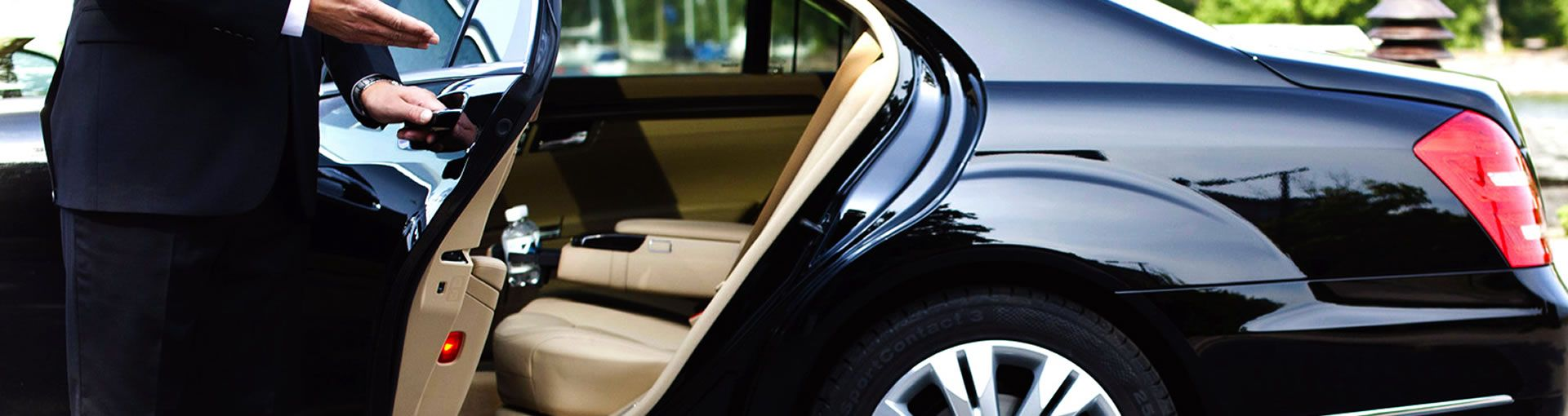###### Hourly Rates  are offered with two potential itinerary scenarios in mind
• The distance between any two points in the itinerary exceeds 50 miles.  Then the rates will be a
• combination of DISTANCE BASED and hourly. The hourly rates are then calculated based on
• wait time combined with time between any two points less than 50 miles distance.
• Itineraries with a mileage less than 50 miles between any two points carries a straight hourly rate
• for the duration of the itinerary.  There is usually a minimum number of hours for this arrangement.
• Rates are based on vehicle type.  See the rate tables per vehicle below.

SEDAN = \$55 per hour and \$3.00 per mile (minimum 4 hours for straight hourly rate)

MINI SUV = \$65 per hour and \$3.50 per mile (minimum 6 hours for straight hourly rate)

ESCALADE SUV = \$75 per hour and \$4.00 per mile (minimum 6 hours for straight hourly rate)

14 PASSENGER VAN = \$90 per hour and \$4.00 per mile (minimum 7 hours for straight hourly rate)

STRETCH LIMOUSINES = \$175 per hour (6 hour minimum)